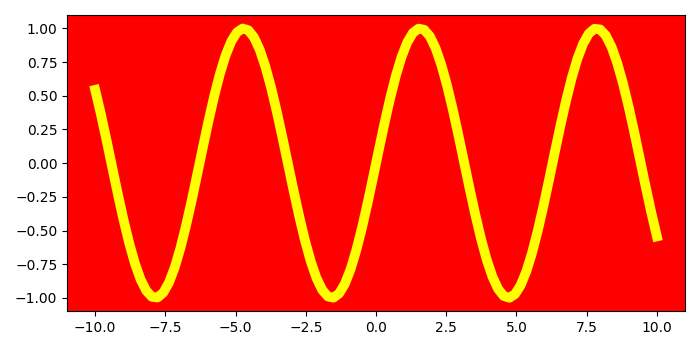# How to change the face color of a plot using Matplotlib?

To change the face color of a plot using matplotlib, we can take the following steps −

• Set the figure size and adjust the padding between and around the subplots.
• Create x and y data points using numpy.
• Create a figure and a set of subplots.
• Plot the x and y data points using plot() method with color=yellow and linewidth=7.
• Set the facecolor of the axes, using set_facecolor().
• To display the figure, use show() method.

## Example

from matplotlib import pyplot as plt
import numpy as np

# Set the figure size
plt.rcParams["figure.figsize"] = [7.00, 3.50]
plt.rcParams["figure.autolayout"] = True

# Create x and y data points
x = np.linspace(-10, 10, 100)
y = np.sin(x)

# Create a figure and set of subplots
fig, ax = plt.subplots()

# Plot the x and y data points with color
ax.plot(x, y, color='yellow', lw=7)

# Set the facecolor
ax.set_facecolor('red')

plt.show()

## Output

It will produce the following output